# Section 5. Classes and Dynamic Memory

Section materials curated by Nick Bowman and Kylie Jue, drawing upon materials from previous quarters.

This week’s section exercise consists of several larger problems that will give you practice with designing classes and working with dynamic array allocation. These problems will help you get practice with the skills that you need for the next assignment, where you will start to implement your very own data structures! As you work on these problems, you may find this Classes and Objects Syntax Sheet to be helpful.

Remember that every week we will also be releasing a Qt Creator project containing starter code and testing infrastructure for that week's section problems. When a problem name is followed by the name of a `.cpp` file, that means you can practice writing the code for that problem in the named file of the Qt Creator project. Here is the zip of the section starter code:

## 1) Circle of Life (`Circle.h/.cpp`)

Topics: Classes

Write a class named `Circle` that stores information about a circle. Your class must implement the following public interface:

``````class Circle {
// constructs a new circle with the given radius
Circle(double r);
// returns the area occupied by the circle
double area() const;
// returns the distance around the circle
double circumference() const;

// returns the radius as a real number
// returns a string representation such as "Circle{radius=2.5}"
string toString() const;
};
``````

You are free to add any private member variables or methods that you think are necessary. It might help you to know that there is a global constant `PI` storing the approximate value of π, roughly `3.14159`.

``````// .h file starts
#pragma once

class Circle {
public:

double area() const;
double circumference() const;
string toString() const;
private:
double r;
}

// .h file ends

// .cpp file starts
#include "Circle.h"

using namespace std;

}

double Circle::area() const{
return PI * r * r;
}

double Circle::circumference() const{
return 2 * PI * r;
}

return r;
}

string Circle::toString() const{
return string("Circle{radius=") + realToString(r) + string("}");
}
// .cpp file ends
``````

## 2) Reciprocate and Divide (`Fraction.h/.cpp`)

Topic: Classes

Consider the following partially-implemented `Fraction` class which can be used to model rational numbers in C++, functionality that is not built-in to the language. If you're interested in the actual source code of the public and private helper methods, feel free to refer to the section starter code.

``````class Fraction {
public:
Fraction();
Fraction(int num, int denom);
void multiply(Fraction f);
double decimal();
int getNumer();
int getDenom();
friend ostream& operator<<(ostream &out, Fraction &frac);
private:
int numer; // stores the numerator of the fraction
int denom; // stores the denominator of the fraction
void reduce(); // simplifies fraction to reduced form
int gcd(int u, int v); // calculates and returns Greatest Common Divisor (GCD) of the two inputs
}
``````

We're going to expand the interface with two additional methods.

Add a public method named `reciprocal` to the `Fraction` class which converts the fraction to its reciprocal (note that by definition the reciprocal of a number `x` is a number `y` such that `xy == 1` holds). You can assume the numerator and denominator will always be non-zero.

Add a public method named `divide` to the `Fraction` class that takes in a `Fraction f` and divides the original `Fraction` by `f`. You can assume the numerator and denominator will always be non-zero.

``````void Fraction::reciprocal() {
int tempDenom = denom;
denom = numer;
numer = tempDenom;
}

void Fraction::divide(Fraction other) {
multiply(Fraction(other.getDenom(), other.getNumer()));
}
``````

## 3) The Notorious RBQ (`RingBufferQueue.h/.cpp`)

Topics: Classes, dynamic arrays

Think back to week 2 when we studied collections. We learned about Queues, a "first-in, first-out" data structure. Today in section, we're going to implement a special type of queue called a Ring Buffer Queue. A Ring Buffer Queue, or RBQ, is implemented by using an underlying array. In our implementation, the capacity is capped; once the array is full, additional elements cannot be added until something is dequeued. Another "interesting" thing about RBQs is that we don't want to shift elements when an element is enqueued or dequeued. Instead, we want to keep track of the front and tail of the Queue. For example, say our queue can hold 5 elements and we enqueue 3 elements: 1, 2, 3. Our queue would look like this: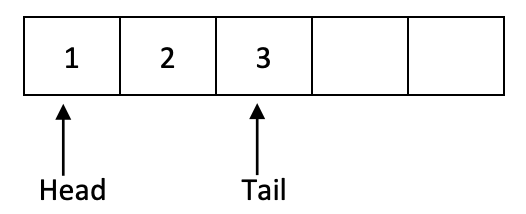If we enqueued two more elements, our queue would then be full: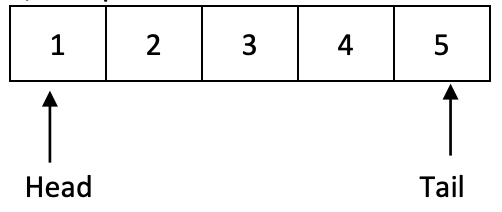At this point, we cannot add any additional elements until we dequeue at least one element. Dequeuing will remove the element at head, and head will move onto the next element. If we dequeue 2 elements, our queue will look like this: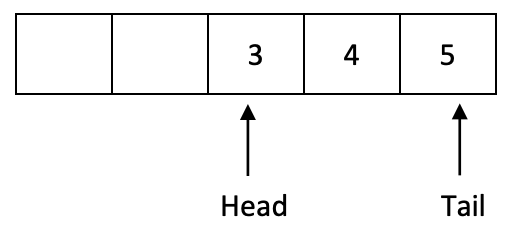Now there's room to add more elements! Since we still don't want to shift any elements, adding an additional element will wrap around. So, if we enqueue an element, our queue will look like this: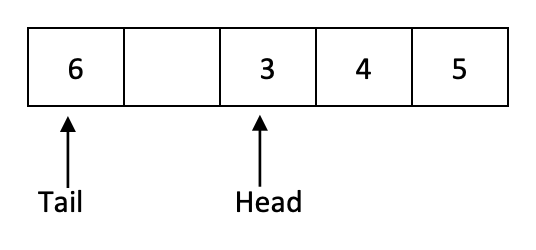Notice that the tail's index is less than the head's index!

Your job is to implement a `RingBufferQueue` class. Your class should have the following public methods:

Method Description
`void enqueue(int elem)` Enqueues `elem` if the queue has room; throws an error if queue is full
`int dequeue()` Returns and removes the element at the front of the queue; throws a string exception if queue is empty
`int peek()` Returns element at the front of the queue; throws a string exception if queue is empty
`bool isEmpty()` Returns `true` if queue is empty and `false` otherwise
`bool isFull()` Returns `true` if queue is full and `false` otherwise
`int size()` Returns number of elements in the queue

You are welcome to add any private methods or fields that are necessary.

It can be hard to know where to start when writing an entire class, so we've given you this breakdown:

1. Start by identifying the private fields you will need, then write the constructor and destructor to initialize the fields and do any cleanup, if necessary. Questions to think about:
• Is it easier to keep track of head and tail (as pictured in the diagrams above)? Or would it be better to track head and size?
2. Write `isEmpty()`, `isFull()`, `size()`, and `peek()`. Questions to think about:
• Which of these methods can be const? In general, how do you know when a method can be const?
3. Write `enqueue()` and `dequeue()`. Remember to handle error conditions! Questions to think about:
• Can you call the methods from part 2 to reduce redundancy?
• Would using modular math help with wrapping around?
• Should either of these methods be const?
4. Finally, deal with ostream insertion!

If you want more practice with writing classes, think about how you could modify this class to implement a double-ended queue. (A double-ended queue, or deque, is one where you can enqueue and dequeue from either the front or the back).

`RingBufferQueue.h`

``````#pragma once

#include <iostream>
class RBQueue {
public:
/* Constructs a new empty queue. */
RBQueue();

~RBQueue();

/* Returns true if the queue contains no elements. */
bool isEmpty() const;

/* Returns true if no additional elements can be enqueued. */
bool isFull() const;

/* Returns number of elements in queue. */
int size() const;

/* Adds the given element to back of queue. */
void enqueue(int elem);

/*
* Removes and returns the front element from the queue
* Throws a string exception if the queue is empty.
*/
int dequeue();

/*
* Returns the front element from the queue without removing it.
* Throws a string exception if the queue is empty.
*/
int peek() const;

private:
// member variables (instance variables / fields)
int* _elements;
int _capacity;
int _numUsed;

// by listing this here as a "friend", it can access the private member variables
friend std::ostream& operator <<(std::ostream& out, const RBQueue& queue);
};
``````

`RingBufferQueue.cpp`

``````#include "RingBufferQueue.h"

const int kDefaultCapacity = 10;

using namespace std;

RBQueue::RBQueue() {
_capacity = kDefaultCapacity;
_elements = new int[_capacity];
_numUsed = 0;
}

RBQueue::~RBQueue() {
delete[] _elements;
}

bool RBQueue::isEmpty() const {
return _numUsed == 0;
}

bool RBQueue::isFull() const {
return _numUsed == _capacity;
}

int RBQueue::size() const {
return _numUsed;
}

int RBQueue::peek() const {
if (isEmpty()) {
throw "Can't peek from an empty queue!";
}

}

int RBQueue::dequeue() {
if (isEmpty()) {
throw "Can't dequeue from an empty queue!";
}

_numUsed--;
return front;
}

void RBQueue::enqueue(int elem) {
if (isFull()) {
throw "Can't enqueue to already full queue!";
}

int tail = (_head + _numUsed) % _capacity;
_elements[tail] = elem;
_numUsed++;
}

ostream& operator <<(ostream& out, const RBQueue& queue) {
out << "{";

if (!queue.isEmpty()) {
// we can access the inner '_elements' member variable because
// this operator is declared as a 'friend' of the queue class

for (int i = 1; i < queue._numUsed; i++) {
int index = (queue._head + i) % queue._capacity;
out << ", " << queue._elements[index];
}
}

out << "}";
return out;
}
``````

## 4) Cleaning Up Your Messes

Topics: Dynamic allocation and freeing

Whenever you allocate an array with `new[]`, you need to deallocate it using `delete[]`. It's important when you do so that you only deallocate the array exactly once – deallocating an array zero times causes a memory leak, and deallocating an array multiple times usually causes the program to crash. (Fun fact – deallocating memory twice is called a double free and can lead to security vulnerabilities in your code! Take CS155 for details.)

Below are three code snippets. Trace through each snippet and determine whether all memory allocated with `new[]` is correctly deallocated exactly once. If there are any other errors in the program, make sure to report them as well.

Snippet 1

``````int main() {
int* arya = new int;
int* jon = new int;

arya = jon;
jon = arya;

delete[] arya;
delete[] jon;

return 0;
}
``````

Snippet 2

``````int main() {
int* stark = new int;
int* lannister = new int;

delete[] stark;
stark = lannister;

delete[] stark;
return 0;
}
``````

Snippet 3

``````int main() {
int* tyrell = new int;
int* arryn = tyrell;

delete[] tyrell;
delete[] arryn;

return 0;
}
``````

The first piece of code has two errors in it. First, the line

`arya = jon`;

causes a memory leak, because there is no longer a way to deallocate the array of three elements allocated in the first line. Second, since both arya and jon point to the same array, the last two lines will cause an error.

The second piece of code is perfectly fine. Even though we execute

`delete[] stark`;

twice, the array referred to each time is different. Remember that you delete arrays, not pointers.

Finally, the last piece of code has a double-delete in it, because the pointers referred to in the last two lines point to the same array.

## 5) Creative Destruction

Topics: Constructors and Destructors

Constructors and destructors are unusual functions in that they're called automatically in many contexts and usually aren't written explicitly. To help build an intuition for when constructors and destructors are called, trace through the execution of this program and list all times when a constructor or destructor is called.

``````/* Prints the elements of a stack from the bottom of the stack up to the top
* of the stack. To do this, we transfer the elements from the stack to a
* second stack (reversing the order of the elements), then print out the
* contents of that stack.
*/
void printStack(Stack<int> toPrint) {
Stack<int> temp;
while (!toPrint.isEmpty()) {
temp.push(toPrint.pop());
}

while (!temp.isEmpty()) {
cout << temp.pop() << endl;
}
}

int main() {
Stack<int> elems;
for (int i = 0; i < 10; i++) {
elems.push(i);
}

printStack(elems);
return 0;
}
``````

The ordering is as follows:

• A constructor is called when elem is declared in main.
• A constructor is then called to set `toPrint` equal to a copy of `elem`.
• A constructor is then called to initialize the `temp` variable in `printStack`.
• When `printStack` exits, a destructor is called to clean up the `temp` variable.
• Also when `printStack` exits, a destructor is called to clean up the `toPrint` variable.
• When `main` exits, a destructor is called to clean up the `elem` variable.

Content for the following two questions is covered in the lecture on Friday, July 30

## 6) Min Heap

Topics: Heaps

We have implemented the Priority Queue ADT using a binary min-heap. Draw a diagram of the heap’s tree structure that results from inserting the following priority values in the order given: `25, 37, 28, 12, 30, 3`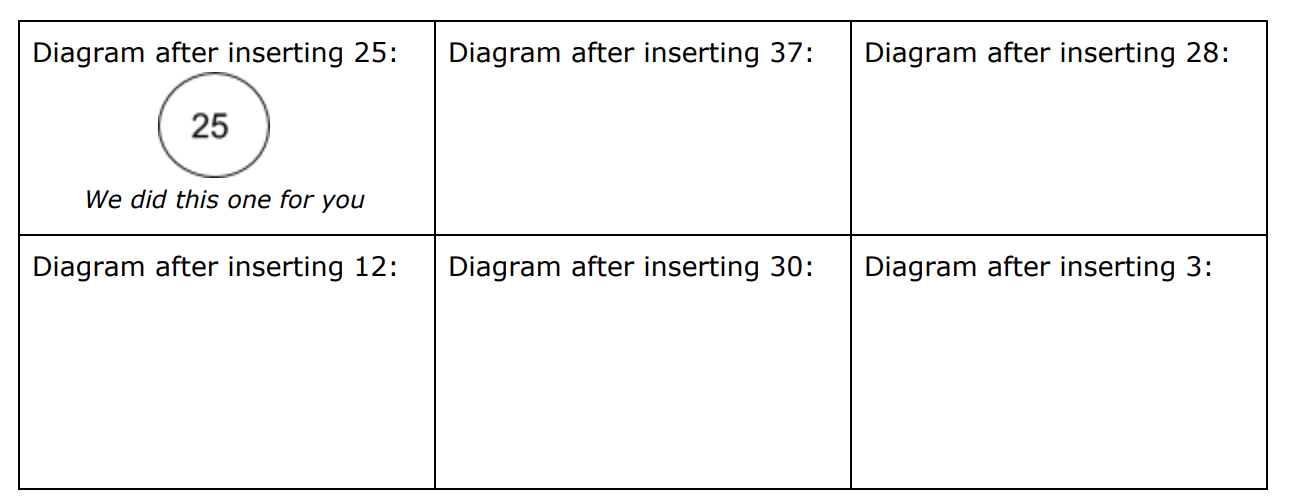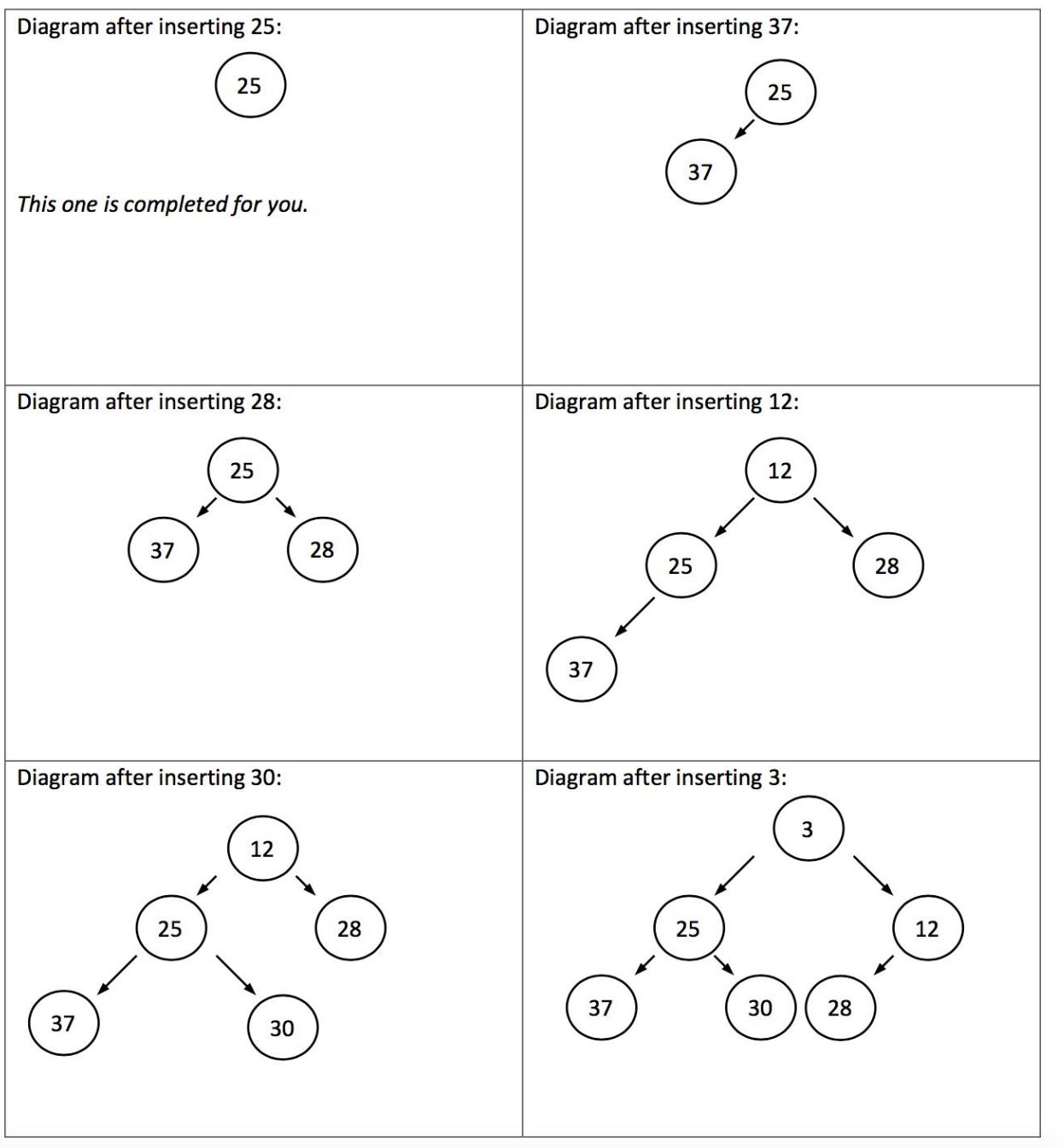## 7) Max Heap

Topics: Heaps

You have a PriorityQueue class which treats higher (larger integer value) priority elements as frontmost. The internal implementation of the class is a binary max-heap stored in an unfilled array. The initial allocation of the array is for capacity 5 and the array capacity is doubled when asked to enqueue to a queue which is full. You are going to trace the operation of enqueuing and dequeuing elements from the priority queue. You can sketch as a tree if you prefer when working it out, but your final answer should be based on a representation of the underlying array that supports the heap.

(a) Show the contents of the internal array after these elements are enqueued to an empty priority queue in this order. Each element has a string value and a priority in parenthesis.

`Red(8), Blue(33), Green(29), Purple(42), Orange(20), Yellow(22), Indigo(10), Teal(21)`

(b) Dequeue is called twice on the priority queue. Which two values are removed?

(c) Show the contents of the internal array after the above two elements have been dequeued

(a) Color names are abbreviated

```

elements:
P(42)
B(33)
G(29)
T(21)
O(20)
Y(22)
I(10)
R(8)
?
?

index:
0
1
2
3
4
5
6
7
8
9

```

(b) Purple(42) and Blue(33)

(c)

```

elements:
G(29)
T(21)
Y(22)
R(8)
O(20)
I(10)
?
?
?
?

index:
0
1
2
3
4
5
6
7
8
9

```